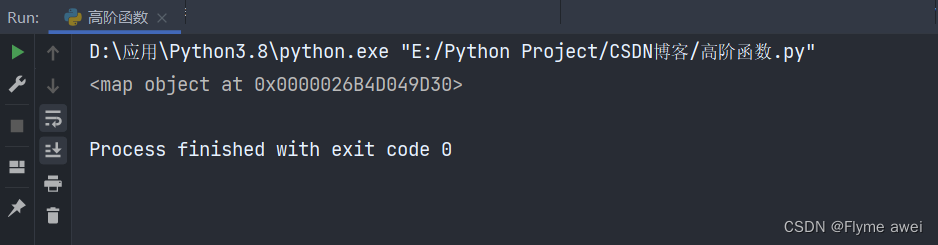> 文档中心 > 【Python函数式编程③】——Python高阶函数 map、reduce、filter

# Python函数式编程–Python高阶函数### 文章目录

• Python函数式编程--Python高阶函数
• @[TOC](文章目录)
• 一、函数式编程
• 1.什么是函数？
• 2.什么是函数式编程？
• 3.高阶函数
• 二、map 函数
• 三、reduce 函数
• 四、filter 函数
• 五、 max 和 min
• 六、sorted 函数
• 七、sum 函数

## 一、函数式编程

### 2.什么是函数式编程？

Python对函数对函数式编程提供了部分支持。由于Python允许使用变量，因此，Python不是纯函数式编程语言。

``>>> abs(-5) 5>>> abs <built-in function abs>``

``>>> i = abs(-5) >>> i 5>>> f = abs >>> f <built-in function abs> >>> f(-5) 5``

``>>> abs = 5 >>> abs(-5) Traceback (most recent call last): File "", line 1, in <module>   abs(-5) TypeError: 'int' object is not callable >>> abs 5``

TypeError: ‘int’ object is not callable 提示，类型错误，int类型是不可以被调用的，我们看到，abs这个变量被赋值5，然后使用abs(-5)调动函数，发现异常，此时abs变量指向的不是函数，而是一个int类型的 5 ，实际上，我们工作或是开发中写代码，是不能这么写的，由此可以看出函数名其实就是变量

``import builtins builtins.abs = 10``

### 3.高阶函数

``# -*- coding: UTF-8 -*- # 文件名：fun_a.py ef fun(i):    return i * 2def total(x, y, fun):    return fun(x) + fun(y)add_sum = total(1, 2, fun)print(add_sum)  # 6``

## 二、map 函数

map（）函数，把一个可迭代对象中的每一个元素换成一个新的对象，最终返回一个迭代器

``map(func, *iterables) --> map object``

``"""func：代表传入参数为函数，这里的函数指定指向函数的函数名*iterables：代表参数指定的可迭代的返回值：返回处理好的数据 map()函数：是将传入的func函数作用于，可迭代的数据里#面每个元素，并将处理好的新的结果返回"""``

``def fun_a(x):return x*10list_a = map(fun_a, [1, 2, 3, 4, 5])list(lst_a)# [10, 20, 30, 40, 50]``

map() 传入的第一个参数是 fun_a ，即函数对象本身。由于结果 list_a 是一个 Iterator ， Iterator 是惰性序列，因此通过 list() 函数让它把整个序列都计算出来并返回一个list。

``list(map(str, [1, 2, 3, 4, 5]))# ['1', '2', '3', '4', '5']``

``# map()函数，把一个可迭代对象中的每一个元素换成一个新的对象，最终返回一个迭代器lst = [1, 2, 3, 4, 5]new_lst = map(lambda x: x**2, lst)``
``print(new_lst)````for i in new_lst:    print(i, end=' ')    # 1 4 9 16 25 ``

## 三、reduce 函数

reduce（）函数，把一个可迭代对象中的每个元素做聚合处理，最终返回一个聚合之后的值

reduce函数是在 functools模块里面的

``from functools import reduce reduce(function, sequence[, initial]) -> value``

``"""function:一个有两个参数的函数 sequence:是一个序列，是一些数据的集合，或者是一组数据，可迭代对象 initial：可选，初始参数 返回值：返回函数计算的结果 reduce()函数,使用function函数（有两个参数）先对集合中的sequence第 1、2 个元素进行操作，如果存在 initial参数，则将会以sequence中的第一个元素和initial作为参数，用作调用，得到的结果再与sequence中的 下一个数据用 function 函数运算，最后得到一个结果。"""``

``from functools import reduce list_a = [1, 2, 3, 4, 5] def fun_b(x, y): return x + y print(reduce(fun_b, list_a)) # 运算结果如下   15``

## 四、filter 函数

filter（）函数 把一个可迭代对象中的元素做过滤操作，如果func返回值为True则留下。否则过滤掉

Python内建的 filter() 函数用于过滤序列，和 map() 类似， filter() 也接收一个函数和一个序列

``filter(function, iterable)``

``"""function：判断函数。 iterable：序列，（可迭代对象）。 返回值：返回列表 filter函数，序列（可迭代对象）的每个元素作为参数传递给函数进行判断，然后返回 True 或 False，最后将返 回 True 的元素放到新列表中"""``

``def not_odd(num):    return num % 2 == 0new_lst = filter(not_odd, [1, 2, 3, 4, 5, 6, 7, 8, 9, 10])print(list(new_lst))# 运算结果如下：# [2, 4, 6, 8, 10]``

### 1.用filter来计算素数

``def oddnum():    n = 1    while True: n += 2 yield ``

``def undivisible(n):    return lambda x: x % n > 0``

``def primes():    yield 2    it = oddnum()    while True: n = next(it) yield n it = filter(undivisible(n), it)``

``# 打印100以内的素数for i in primes():    if i < 100: print(i)    else: break ``

## 五、 max 和 min

``def max(*args, key=None): # known special case of max    """    max(iterable, *[, default=obj, key=func]) -> value    max(arg1, arg2, *args, *[, key=func]) -> value With a single iterable argument, return its biggest item. The    default keyword-only argument specifies an object to return if    the provided iterable is empty.    With two or more arguments, return the largest argument.    """    passdef min(*args, key=None): # known special case of min    """    min(iterable, *[, default=obj, key=func]) -> value    min(arg1, arg2, *args, *[, key=func]) -> value With a single iterable argument, return its smallest item. The    default keyword-only argument specifies an object to return if    the provided iterable is empty.    With two or more arguments, return the smallest argument.    """    pass``
``print(max(1, 2, 3, 4, 5, 6, 7, 8, 9, 10))# 10print(min(1, 2, 3, 4, 5, 6, 7, 8, 9, 10))# 1``

## 六、sorted 函数

sorted（）函数 把一个可迭代对象里面的每个元素做排序

``def sorted(*args, **kwargs): # real signature unknown    """    Return a new list containing all items from the iterable in ascending order. A custom key function can be supplied to customize the sort order, and the    reverse flag can be set to request the result in descending order.    """    pass``
``print(sorted([1, 5, 2, 3, 10, 6, 8]))# 输出结果如下# [1, 2, 3, 5, 6, 8, 10]print(sorted([1, 5, 2, 3, 10, 6, 8], reverse=True))# 输出结果如下#[10, 8, 6, 5, 3, 2, 1]``

## 七、sum 函数

sum（）函数 求和

``def sum(*args, **kwargs): # real signature unknown    """    Return the sum of a 'start' value (default: 0) plus an iterable of numbers When the iterable is empty, return the start value.    This function is intended specifically for use with numeric values and may    reject non-numeric types.    """    pass``
``print(sum([10, 8, 6, 5, 3, 2, 1]))# 35``